### 13.4.3.1 Incorporating velocity constraints

The Lagrangian formulation of Section 13.4.1 can be extended to allow additional constraints placed on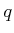and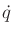. This is very powerful for developing state transition equations for robots that have closed kinematic chains or wheeled bodies. If there are closed chains, then the configurations may be restricted to lie in a subset of. If a parameterization of the solution set is possible, thencan be redefined over the reduced C-space. This is usually not possible, however, because such a parametrization is difficult to obtain, as mentioned in Section 4.4. If there are wheels or other contact-based constraints, such as those in Section 13.1.3, then extra constraints onandexist. Dynamics can be incorporated into the models of Section 13.1 by extending the Euler-Lagrange equation.

The coming method will be based on Lagrange multipliers. Recall from standard calculus that to optimize a function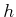defined over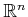, subject to an implicit constraint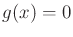, it is sufficient to consider only the extrema of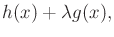(13.162)

in which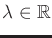represents a Lagrange multiplier . The extrema are found by solving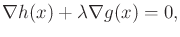(13.163)

which expressesequations of the form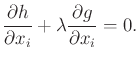(13.164)

The same principle applies for handling velocity constraints on.

Suppose that there are velocity constraints onas considered in Section 13.1. Consider implicit constraints, in which there areequations of the form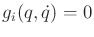forfromto. Parametric constraints can be handled as a special case of implicit constraints by writing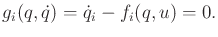(13.165)

For any constraints that contain actions, no extra difficulties arise. Eachis treated as a constant in the following analysis. Therefore, action variables will not be explicitly named in the expressions.

As before, assume time-invariant dynamics (see  for the time-varying case). Starting with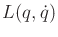defined using (13.130), let the new criterion be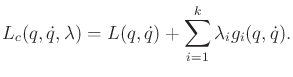(13.166)

A functional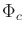is defined by substitutingfor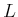in (13.114).

The extremals ofare given byequations,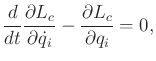(13.167)

andequations,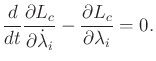(13.168)

The justification for this is the same as for (13.124), except now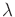is included. The equations of (13.168) are equivalent to the constraints. The first term of each is zero because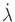does not appear in the constraints, which reduces them to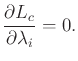(13.169)

This already follows from the constraints on extremals ofand the constraints. In (13.167), there areequations in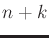unknowns. TheLagrange multipliers can be eliminated by using theconstraints. This corresponds to Lagrange multiplier elimination in standard constrained optimization .

The expressions in (13.167) and the constraints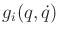may be quite complicated, which makes the determination of a state transition equation challenging. General forms are given in Section 3.8 of . An important special case will be considered here. Suppose that the constraints are Pfaffian,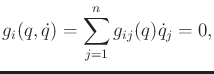(13.170)

as introduced in Section 13.1. This includes the nonholonomic velocity constraints due to wheeled vehicles, which were presented in Section 13.1.2. Furthermore, this includes the special case of constraints of the form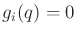, which models closed kinematic chains. Such constraints can be differentiated with respect to time to obtain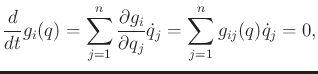(13.171)

which is in the Pfaffian form. This enables the dynamics of closed chains, considered in Section 4.4, to be expressed without even having a parametrization of the subset ofthat satisfies the closure constraints. Starting in implicit form, differentiation is required to convert them into the Pfaffian form.

For the important case of Pfaffian constraints, (13.167) simplifies to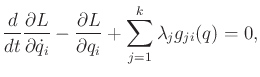(13.172)

The Pfaffian constraints can be used to eliminate the Lagrange multipliers, if desired. Note that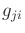represents theth term of the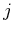th Pfaffian constraint. An action variablecan be placed on the right side of each constraint, if desired.

Equation (13.172) often appears instead as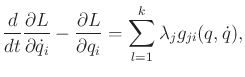(13.173)

which is an alternative but equivalent expression of constraints because the Lagrange multipliers can be negated without affecting the existence of extremals. In this case, a nice interpretation due to D'Alembert can be given. Expressions that appear on the right of (13.173) can be considered as actions, as mentioned in Section 13.4.1. As stated previously, such actions are called generalized forces in mechanics. The principle of virtual work is obtained by integrating the reaction forces needed to maintain the constraints. These reaction forces are precisely given on the right side of (13.173). Due to the cancellation of forces, no true work is done by the constraints (if there is no friction).

Example 13..14 (A Particle on a Sphere)   Suppose that a particle travels on a unit sphere without friction or gravity. Let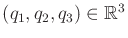denote the position of the point. The Lagrangian function is the kinetic energy,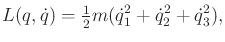(13.174)

in which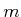is the particle mass. For simplicity, assume that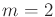.

The constraint that the particle must travel on a sphere yields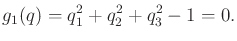(13.175)

This can be put into Pfaffian form by time differentiation to obtain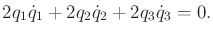(13.176)

Since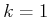, there is a single Lagrange multiplier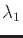. Applying (13.172) yields three equations,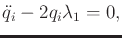(13.177)

forfromto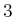. The generic form of the solution is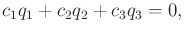(13.178)

in which the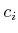are real-valued constants that can be determined from the initial position of the particle. This represents the equation of a plane through the origin. The intersection of the plane with the sphere is a great circle. This implies that the particle moves between two points by traveling along the great circle. These are the shortest paths (geodesics) on the sphere.The general forms in Section 13.4.2 can be extended to the constrained case. For example, (13.142) generalizes to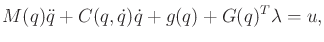(13.179)

in which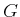is a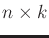matrix that represents all of thePfaffian coefficients. In this case, the Lagrange multipliers can be computed as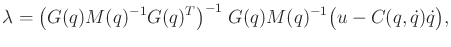(13.180)

assumingis time-invariant.

The phase transition equation can be determined in the usual way by performing the required differentiations, defining the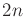phase variables, and solving for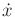. The result generalizes (13.148).

Steven M LaValle 2020-08-14